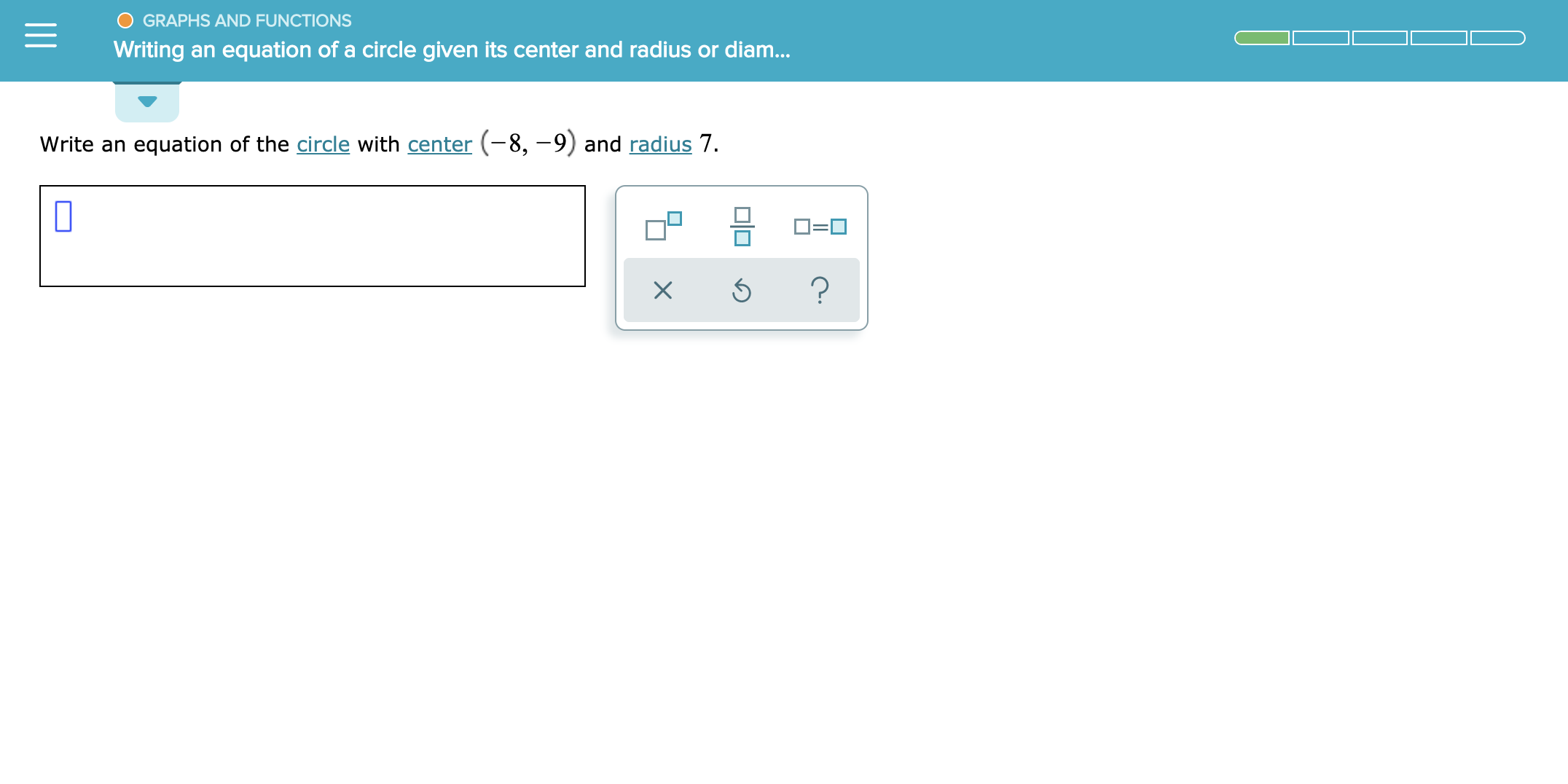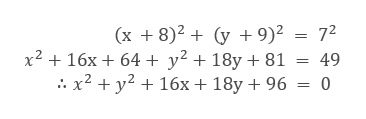# O GRAPHS AND FUNCTIONSWriting an equation of a circle given its center and radius or diam...Write an equation of the circle with center (-8,-9) and radius 7.?

Question
12 views

See attachmenthelp_outlineImage TranscriptioncloseO GRAPHS AND FUNCTIONS Writing an equation of a circle given its center and radius or diam... Write an equation of the circle with center (-8,-9) and radius 7. ? fullscreen
check_circle

Step 1

Given a circle with center (-8, -9) and radius as 7.

To Find: Equation of circle.

Step 2

Comparing with the general form we have,

Step 3

Substitute the value for h, k and r ...help_outlineImage Transcriptionclose(x 8)2y 9)2 = 72 x216x 64 y2 + 18y + 81 = 49 x2y2 + 16x+ 18y + 96 = 0 fullscreen

### Want to see the full answer?

See Solution

#### Want to see this answer and more?

Solutions are written by subject experts who are available 24/7. Questions are typically answered within 1 hour.*

See Solution
*Response times may vary by subject and question.
Tagged in

### Other